## Saturday, June 3, 2017

### Where am I?

I have not posted here in a long time, but continue to be active with mathematics.  If you'd like to see more mathematical imagery and read about it, please visit my G+ feed or check my website.  Maybe I'll be back here some day, maybe not.

## Friday, September 6, 2013

### The dual {5,3,4} and {4,3,5}

I've been collaborating with +Henry Segerman to create some physical models of hyperbolic honeycombs, and here is one of the first we made, the {5,3,4}.  If you would like to order one of these for yourself, information is at the bottom of this post.

What is a honeycomb?
It is when you take a bunch of polyhedra and pack them together with no gaps.  The polyhedra in a honeycomb are called "cells".  This particular honeycomb is a perfectly regular repeating pattern of dodecahedra, four surrounding each edge.  For this reason, it is also called the Order-4 Dodecahedral Honeycomb.

Why is it hyperbolic?
Hyperbolic geometry is a space where the angles of a triangle add up to less than 180 degrees.  The other kinds of geometries are Euclidean (angles add to 180 degrees) and spherical (angles add to more than 180 degrees).  If you try to pack four dodecahedra around an edge in Euclidean or spherical space, they don't fit because angles are too big.  But in hyperbolic geometry, you can make them fit.

Hyperbolic space can't fit into our normal Euclidean space, so this is a model of hyperbolic space called the Poincaré Ball model.  The infinity of hyperbolic space is compressed to the size of a ball, and as you might imagine, that results in some distortion.  Dodecahedra appear smaller and smaller as they approach the spherical surface of the ball, but the dodecahedra are all identical in hyperbolic space.  Although visually warped, the ball model preserves all angles: it is "conformal".

Our prints render edges of the honeycomb, and we've scaled them accurately (verses making them constant width).  For this reason, the edges appear thinner towards the ball surface.  The edge shrinking dictates how much of the honeycomb we can include, because as you add more layers of cells, edges eventually become so thin that a printed model would be too fragile.

What does the cryptic "{5,3,4}" mean?
That is something called the Schläfli symbol, and it is wonderfully descriptive (and recursive!).  We can start at the left and work our way to the right to understand the meaning and build up a honeycomb.  The Schläfli symbol for a pentagon is {5} because it has 5 sides.  The symbol for a dodecahedron is {5,3}, which means that 3 pentagons meet at every vertex.  So the first number specifies a particular polygon and the second number describes how to connect up those polygons around a vertex.

The third number continues the trend.  It describes how to connect up cells specified by the first two numbers.  So the 4 at the end of {5,3,4} means that four dodecahedra surround each edge.  Each time we add a number to a Schläfli symbol, we end up describing an object of one higher dimension: first polygons, then polyehedra, then polytopes...

Something else is hidden in the Schläfli symbol of our honeycomb: the last two numbers taken together have meaning.  For the {5,3,4}, we're talking about {3,4}.  Remember {3} represents a triangle, and {3,4} means that four triangles meet at every vertex.  This is the octahedron.

How is the octahedron is meaningful for this honeycomb?  It is the the vertex figure, which describes the geometry of how dodecahedra meet at each vertex in the honeycomb.  Eight dodecahedra meet at every vertex, one for each face of the octahedron.

What a crazy amount of information compressed into three numbers!

There are four regular honeycombs in hyperbolic space (and eleven more if you loosen the definition a little).  Each of these has a unique Schläfli symbol, and so the symbol is a great way to quickly understand how a particular honeycomb is built up.  The symbols also work for honeycombs in Euclidean and spherical space.

Now let's check out the {4,3,5}

Can you break down the symbol to understand how this one is built up?  What polygon lives throughout?  What are the polyhedral cells?  How many cells fit around an edge?  What is the vertex figure?

The {4,3,5} is the "dual" of the {5,3,4}, so locations of all the element types are exchanged.  Where there are cells, polygons, edges, and vertices in one, you respectively have vertices, edges, polygons, and cells in the other.  And maybe you just noticed something else about the Schläfli symbol... dual objects have reversed symbols.  Self-dual objects have palindromic Schläfli symbols!

You can order your own prints of both these models (and more exotic honeycombs) at my shapeways shop.

If you'd like to dig more, see Coxeter's paper, "Regular Honeycombs in Hyperbolic Space", available in Chapter 10 of the book "The Beauty of Geometry" or online here.

## Sunday, July 1, 2012

### Recursive Circle Inversions

I played around with recursive circle inversions this weekend.  A circle inversion is like a reflection in a mirror, except the mirror is curved.  I start out with a small set of tangent circles, then repeatedly reflect all the circles in each other.  The fractal results can be pretty dramatic...

Lots of {3,infinity} hyperbolic tessellations floating around!

If you like these images, I highly recommend the book Indra's Pearls.

## Tuesday, December 13, 2011

### Dodecahedron in Disguise

I like this image, created while experimenting with MagicTile last night.

## Sunday, December 11, 2011

### Interference Patterns

Edmund Harris recently posted some beautiful pictures created with modular arithmetic. I was surprised and intrigued to see this one, because it so closely resembled an image I made some years ago without thinking about modular arithmetic at all. I dug through my disorganized backups, and finally found it from circa 2004.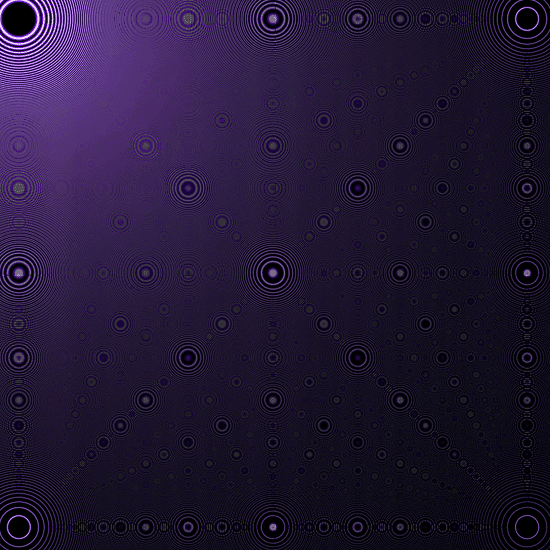These images don't resize well. The scaling can both remove structure and produce artifacts that are not in the original, so here is an example detail portion of the full image.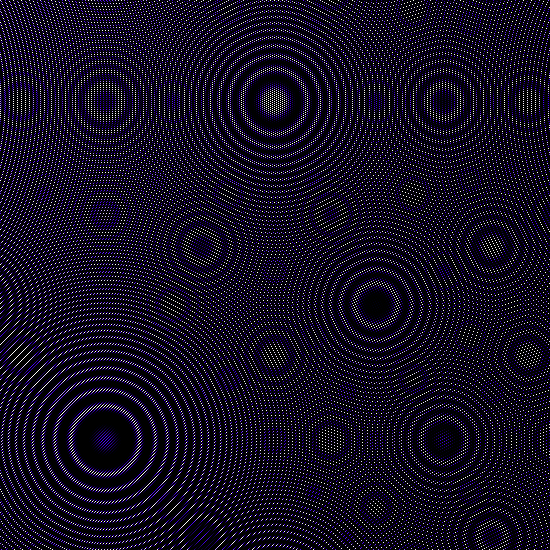These images are fractal looking, with repeating patterns at smaller scales. You can see the integers and fractions living in there. Perhaps cooler, the smaller repetitions seem to exist on a "higher level" than the basic repeating unit. They float along the patterns below, having a sort of ethereal existence.

If Edmund used modular arithmetic, how was my image made? Consider the function sin(x2). Here is a plot of it in the range of 0 to 15. You can see the frequency of the oscillations is increasing quickly with larger x.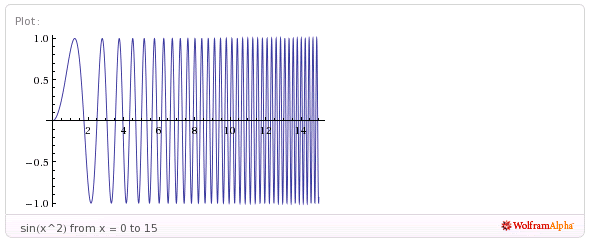Now consider sampling this function at discrete values of x in the larger range of 0 to 1000. When you get to higher values of x, this continuous function is oscillating back and forth a huge number of times between each successive value of x. Is there a pattern to be seen in the discretely sampled output values?

Finding a pattern on a 1D line is not so easy, but you can plot discrete values of sin(d2) on a 2D grid, where d is the distance of an image pixel to the origin. When the function value for each discrete pixel is calculated and plotted as a color, voila! The image appears, and the patterns undeniably jump out.

At the time, I attributed the effect to a Moire-like phenomenon, with an interference between the discrete grid of pixels and the continuous function. It was neat to read Edmund's explanation that modular arithmetic is behind the scenes. Maybe modular arithmetic is part of the magic behind Moire patterns?

I experimented with other functions as well, and here is a favorite. I love that it has both elliptical and hyperbolic patterns in it, and the transitioning between the two.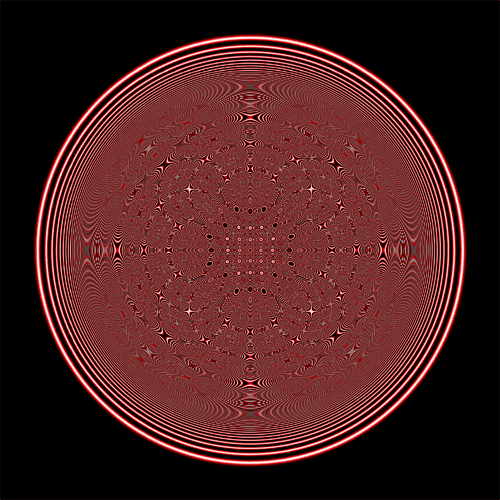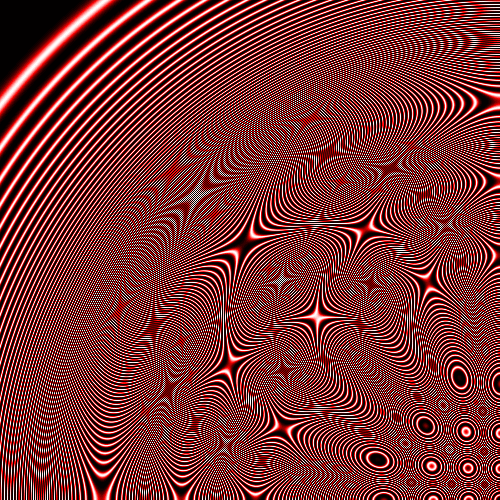## Tuesday, May 17, 2011

### Three Different Views of the Same Tiling

Here are three images of the same {4,6} tiling. The "{4,6}" notation is called the Schläfli symbol. The 4 means it is a tiling of squares. The 6 means that 6 squares meet at every vertex. Check it in the pictures!

A view centered on a square:
A view centered on an edge:
A view centered on a vertex:
I bet you noticed that the tiling is checkerboarded (hyperbolic chess anyone?). Can you figure out what property is required to allow a tiling to be checkerboarded? If so, let me know in the comments!

## Sunday, April 17, 2011

It's highly likely you've seen a geodesic dome before.After briefly starting to optimize triangle counts for textures in MagicTile, I had a fun realization. The triangle patterns sparked the idea that there could be a precise hyperbolic analogue to a geodesic dome. I was compelled into the diversion, and with minor code changes made some pretty pictures of "geodesic saddles". (That seems like a nice name for these objects anyway.) Alas, my intended optimizations are yet to be done, but at least I can present this geodesic saddle based on the {3,7} tiling :)Can you find some of the "knots"? That may not be the proper term, but I mean those rare points in the saddle where seven triangles meet at a vertex instead of six. On a geodesic dome, which is usually based on the spherical {3,5} tiling (aka icosahedron), the analogous points are the rare vertices where five triangles meet instead of six. I find knots easier to spot on a geodesic saddle derived from a {3,9} tiling.Geodesic domes and saddles are generated by taking the tiles in a triangular tiling and subdividing each of them into smaller triangles. Hence, triangular numbers make a cameo in the calculations. For these pictures, I chose to subdivide the original triangles with eight new triangles per side.

But there was one thing that tripped me up quite a bit. I began by mistakenly thinking I could subdivide the triangle edges of the original tiling equally, and then interpolate interior points thereafter. As much as I tried, things just wouldn't line up quite right, and I wasn't seeing the geodesics that I expected. It turns out that all the small triangle edges have varying lengths, something that is also true for a geodesic dome. Compare the proper {3,9} geodesic saddle above with the waviness of an incorrect effort.I've been showing these pictures in the Poincare Disk, and I don't have unprojected renderings at the moment. But despite this, one thing is certain - a portion of geodesic saddle would make for a unique and fantastic jungle gym!